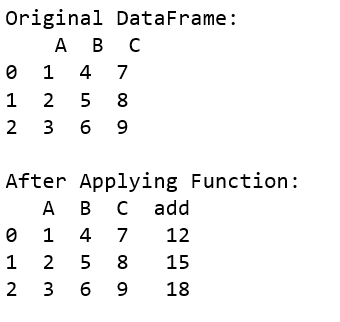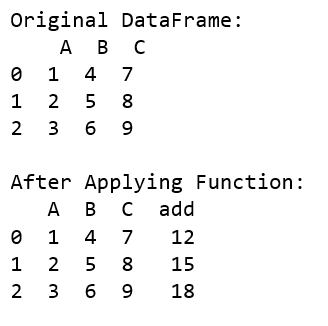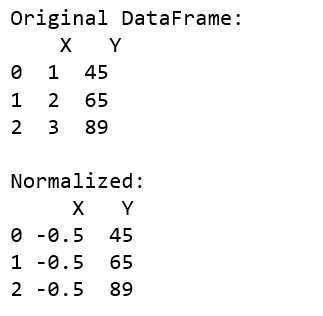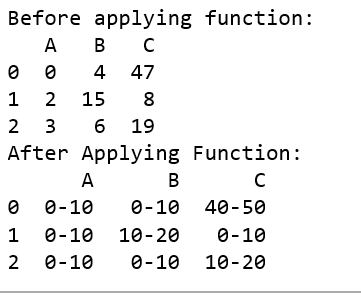GeeksforGeeks App
Open AppBrowser
Continue

# Apply function to every row in a Pandas DataFrame

Python is a great language for performing data analysis tasks. It provides with a huge amount of Classes and function which help in analyzing and manipulating data in an easier way.
One can use apply() function in order to apply function to every row in given dataframe. Let’s see the ways we can do this task.
Example #1:

## Python3

 `# Import pandas package``import` `pandas as pd` `# Function to add``def` `add(a, b, c):``    ``return` `a ``+` `b ``+` `c` `def` `main():``    ` `    ``# create a dictionary with``    ``# three fields each``    ``data ``=` `{``            ``'A'``:[``1``, ``2``, ``3``],``            ``'B'``:[``4``, ``5``, ``6``],``            ``'C'``:[``7``, ``8``, ``9``] }``    ` `    ``# Convert the dictionary into DataFrame``    ``df ``=` `pd.DataFrame(data)``    ``print``(``"Original DataFrame:\n"``, df)``    ` `    ``df[``'add'``] ``=` `df.``apply``(``lambda` `row : add(row[``'A'``],``                     ``row[``'B'``], row[``'C'``]), axis ``=` `1``)`` ` `    ``print``(``'\nAfter Applying Function: '``)``    ``# printing the new dataframe``    ``print``(df)`` ` `if` `__name__ ``=``=` `'__main__'``:``    ``main()`

Output:Example #2:
You can use the numpy function as the parameters to the dataframe as well.

## Python3

 `import` `pandas as pd``import` `numpy as np`` ` `def` `main():``    ` `    ``# create a dictionary with``    ``# five fields each``    ``data ``=` `{``            ``'A'``:[``1``, ``2``, ``3``],``            ``'B'``:[``4``, ``5``, ``6``],``            ``'C'``:[``7``, ``8``, ``9``] }``    ` `    ``# Convert the dictionary into DataFrame``    ``df ``=` `pd.DataFrame(data)``    ``print``(``"Original DataFrame:\n"``, df)``    ` `    ``# applying function to each row in the dataframe``    ``# and storing result in a new column``    ``df[``'add'``] ``=` `df.``apply``(np.``sum``, axis ``=` `1``)`` ` `    ``print``(``'\nAfter Applying Function: '``)``    ``# printing the new dataframe``    ``print``(df)`` ` `if` `__name__ ``=``=` `'__main__'``:``    ``main()`

Output:Example #3: Normalising Data

## Python3

 `# Import pandas package``import` `pandas as pd` `def` `normalize(x, y):``    ``x_new ``=` `((x ``-` `np.mean([x, y])) ``/``             ``(``max``(x, y) ``-` `min``(x, y)))``    ` `    ``# print(x_new)``    ``return` `x_new` `def` `main():``    ` `    ``# create a dictionary with three fields each``    ``data ``=` `{``        ``'X'``:[``1``, ``2``, ``3``],``        ``'Y'``:[``45``, ``65``, ``89``] }``    ` `    ``# Convert the dictionary into DataFrame``    ``df ``=` `pd.DataFrame(data)``    ``print``(``"Original DataFrame:\n"``, df)``    ` `    ``df[``'X'``] ``=` `df.``apply``(``lambda` `row : normalize(row[``'X'``],``                                  ``row[``'Y'``]), axis ``=` `1``)`` ` `    ``print``(``'\nNormalized:'``)``    ``print``(df)`` ` `if` `__name__ ``=``=` `'__main__'``:``    ``main()`

Output:Example #4: Generate range

## Python3

 `import` `pandas as pd``import` `numpy as np`` ` `pd.options.mode.chained_assignment ``=` `None` `# Function to generate range``def` `generate_range(n):``    ` `    ``# printing the range for eg:``    ``# input is 67 output is 60-70``    ``n ``=` `int``(n)``    ` `    ``lower_limit ``=` `n``/``/``10` `*` `10``    ``upper_limit ``=` `lower_limit ``+` `10``    ` `    ``return` `str``(``str``(lower_limit) ``+` `'-'` `+` `str``(upper_limit))``     ` `def` `replace(row):``    ``for` `i, item ``in` `enumerate``(row):``        ` `        ``# updating the value of the row``        ``row[i] ``=` `generate_range(item)``    ``return` `row``         ` ` ` `def` `main():``    ``# create a dictionary with``    ``# three fields each``    ``data ``=` `{``            ``'A'``:[``0``, ``2``, ``3``],``            ``'B'``:[``4``, ``15``, ``6``],``            ``'C'``:[``47``, ``8``, ``19``] }``    ` `    ``# Convert the dictionary into DataFrame``    ``df ``=` `pd.DataFrame(data)`` ` `    ``print``(``'Before applying function: '``)``    ``print``(df)``     ` `    ``# applying function to each row in``    ``# dataframe and storing result in a new column``    ``df ``=` `df.``apply``(``lambda` `row : replace(row))``     ` ` ` `    ``print``(``'After Applying Function: '``)``    ``# printing the new dataframe``    ``print``(df)`` ` `if` `__name__ ``=``=` `'__main__'``:``    ``main()`

Output:My Personal Notes arrow_drop_up